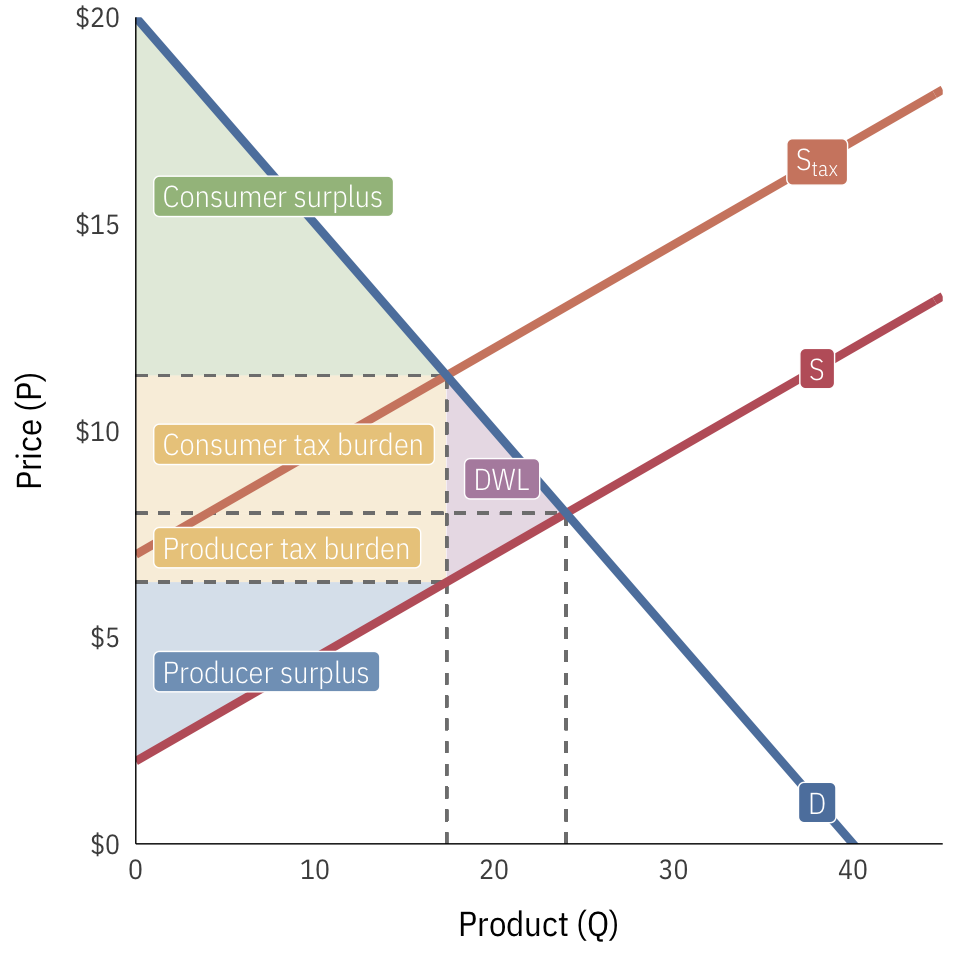# Exam 2 study guide

## Firms and markets

You should understand…

• …how demand curves are derived from consumer willingness to pay
• …the difference between fixed costs and variable costs
• …how to calculate total cost, total revenue, average fixed costs, average variable costs, marginal cost, marginal revenue, and maximum profit
• …that maximum profit occurs where marginal revenue is equal to marginal cost ($MR = MC$)
• …that socially optimal quantity occurs when the demand is equal to the marginal cost ($\text{demand} = MC$)
• …how to calculate elasticity of demand ($-\frac{\Delta Q}{\Delta P} \times \frac{P}{Q}$)
• …what elasticity measures and why it is important in public policy and administration
• …how a single demand curve can have an overall elasticity and different elasticities at each point
• …economies of scale, diseconomies of scale, economies of agglomeration, network effects, and the difference between short-run and long-run costs
• …that market equilibria (i.e. optimal price and quantity) occur at the intersection of supply and demand curves
• …how government-imposed price floors and price ceilings distort market-clearing equilibria
• …and be able to identify the differences between changes in supply/demand and changes in quantity supplied/demanded
• …what consumer and producer surplus represent
• …the relationship between elasticity of supply and/or demand and the size of consumer and producer surplus
• …how taxes impose deadweight loss on society
• …how the burden of taxes depends on the elasticity of supply and/or demand
• …why governments tax and the philosophical and ethical principles behind who should bear the burden of taxes
• …the difference between price-taking and price-making
• …how firms try to gain market power, including monopolies, branding, cost controls, regulation, and switching costs
• …why firms try to gain market power
• …why firms want to price discriminate
• …the consequences of monopolistic production (lower Q and higher P than what would happen under perfect competition; deadweight loss)
• …how governments can regulate monopolies
• …why natural monopolies exist and how governments can induce them to produce at socially optimal levels
• …how firms need to be somewhat anti-competitive and anti-capitalist in order to maximize profits, innovate, and (essentially) be more competitive and capitalist

Guides

Important formulas:

• Demand:

$$P = aQ + b$$

• Total cost:

\begin{aligned} TC = TFC + TVC \\ \text{or a formula using } Q \text{, like} \\ TC = aQ^2 + b \end{aligned}

• Average cost:

$$AC = \frac{TC}{Q}$$

• Marginal cost:

\begin{aligned} MC &= \frac{\Delta TC}{\Delta Q} \\ &\text{or} \\ MC &= \text{First derivative of TC} \\ &= 2aQ \text{ (if } TC = aQ^2 + b) \end{aligned}

• Total revenue:

\begin{aligned} TR &= PQ \\ &\text{or} \\ TR &= (aQ + b)Q \\ &= aQ^2 + bQ \end{aligned}

• Average revenue:

$$AR = \frac{TR}{Q}$$

• Marginal revenue:

\begin{aligned} MR &= \frac{\Delta TR}{\Delta Q} \\ &\text{or} \\ MR &= \text{First derivative of TR} \\ &= 2aQ + b \text{ (if } TR = aQ^2 + bQ) \end{aligned}

• Maximum profit:

$$max(\pi): MC = MR$$

• Price elasticity of demand (see this guide of how to get to $$- \frac{\Delta Q}{\Delta P} \times \frac{P}{Q}$$):

$$\varepsilon = -\frac{\% \text{ change in quantity demand}}{\% \text{ change in price}} = - \frac{\Delta Q}{\Delta P} \times \frac{P}{Q}$$

Important graphs:

• Consumer surplus, producer surplus, tax revenues, tax burdens, and deadweight loss (use algebra and geometry to figure out the areas of the triangles ($\frac{1}{2} \times b \times h$) and rectangles ($l \times w$)):## Institutions, power, and inequality

You should understand…

• …why institutions matter for public administration, policy, and governance
• …the strengths and weaknesses of and the and general differences between three main theoretical approaches to institutions: institutions as rational behavior, institutions as constraints, and institutions as temporary equilibria
• …the difference between informal and formal institutions
• …why informal institutions exert influence over our actions even if they’re not officially codified
• …the difference between self-enforcing, self-reinforcing, and self-undermining institutions
• …the role of path dependency in the emergence of institutions

## Market and government failures

You should understand…

• …the difference between private goods, club goods, common pool resources, and public goods (and how they can be classified as (non)rivalrous and (non)excludable)
• …what a market failure is
• …what a government failure is
• …what public goods are and how governments, the private sector, and informal institutions can address them
• …what common pool resources (CPRs) are and how governments, the private sector, and informal institutions can address them
• …the difference between social and private marginal cost (supply) and marginal benefit (demand)
• …what externalities are and how governments, the private sector, and informal institutions can address them
• …what Coasian bargaining is, when it is advantageous, and why it sometimes fails
• …how cap and trade systems can fix externalities (and when they can’t)
• …how Pigouvian taxes can fix externalities (and when they can’t)
• …how regulation can fix externalities (and when and why it can’t)
• …the difference between income and assets
• …why shared national identity and strong horizontal networks of institutions are important for a country’s social and economic wellbeing
• …how inequitable public policies lead to decreased public goods provision, unequal institutional access, and increased ethnofractionalization
• …why slavery and white supremacy have had lasting institutional impacts on the economic system of today and how government policies have contributed to these consequences (specifically in housing and education)